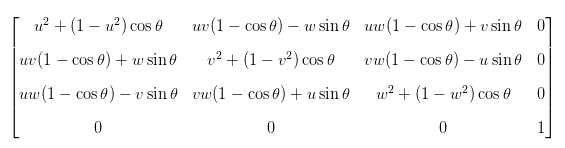# 旋转变换（一）旋转矩阵

### 2. 绕原点二维旋转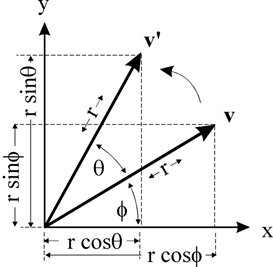x=rcosϕy=rsinϕ$x = r cos\phi \quad y = r sin\phi$
x=rcos(θ+ϕ)y=rsin(θ+ϕ)$x' = r cos(\theta + \phi) \quad y' = rsin(\theta+\phi)$

x=rcosθcosϕrsinθsinϕ$x' = r cos\theta cos\phi - rsin\theta sin\phi$
y=rsinθcosϕ+rcosθsinϕ$y' = rsin\theta cos\phi + r cos\theta sin\phi$

x=xcosθysinθ$x' = xcos\theta - ysin\theta$
y=xsinθ+ycosθ$y' = xsin\theta + ycos\theta$

[xy]=[cosθsinθsinθcosθ][xy]

### 3. 绕任意点的二维旋转

1. 首先将旋转点移动到原点处
2. 执行如2所描述的绕原点的旋转
3. 再将旋转点移回到原来的位置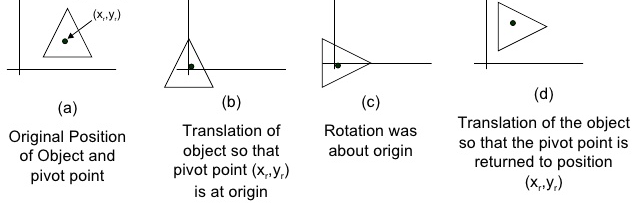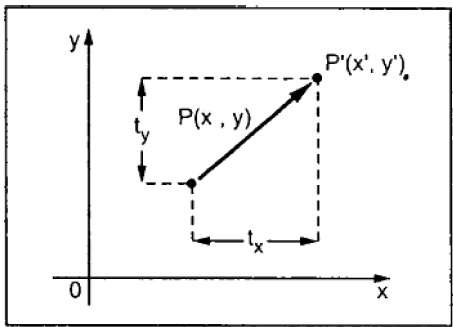x=x+txy=y+ty$x' = x + tx \quad y' = y+ty$

xy1=100010txty1xy1

100010txty1

100010txty1

xy1=cosθsinθ0sinθcosθ0001xy1

M=100010txty1cosθsinθ0sinθcosθ0001100010txty1=cosθsinθ0sinθcosθ0(1cosθ)tx+tysinθ(1cosθ)tytxsinθ1

### 4. 三维基本旋转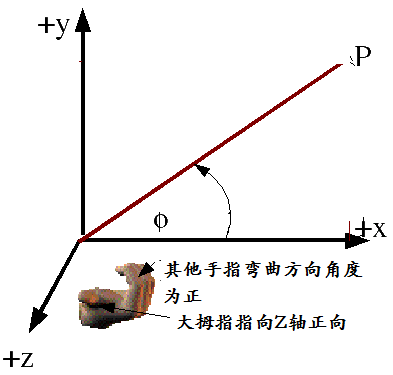#### 4.1 绕X轴的旋转

x=x$\quad x' = x$
y=ycosθzsinθ$\quad y' = ycos\theta - zsin\theta$
z=ysinθ+zcosθ$\quad z' = ysin\theta + zcos\theta$

xyz1=10000cosθsinθ00sinθcosθ00001xyz1

#### 4.2 绕Y轴旋转

x=zsinθ+xcosθ$\quad x' = zsin\theta + xcos\theta$
y=y$\quad y' = y$
z=zcosθxsinθ$\quad z' = zcos\theta-xsin\theta$

xyz1=cosθ0sinθ00100sinθ0cosθ00001xyz1

#### 4.3 绕Z轴旋转

xyz1=cosθsinθ00sinθcosθ0000100001xyz1

#### 4.4 小结

xyz1

zyx1=cosθ0sinθ00100sinθ0cosθ00001zyx1

[cosθsinθsinθcosθ]

### 5. 绕任意轴的三维旋转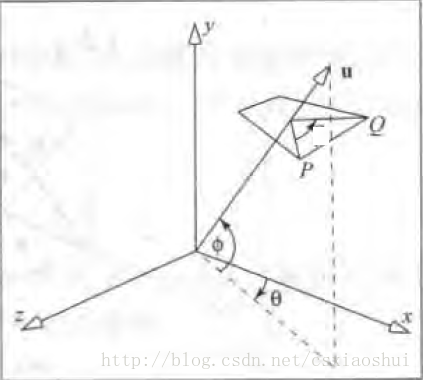P点绕向量u旋转θ$\theta$角，得到点Q，已知P点的坐标和向量u，如何求Q点的坐标。

1. 将旋转轴u绕x轴旋转至xoz平面
2. 将旋转轴u绕y轴旋转至于z轴重合
3. 绕z轴旋转θ$\theta$
4. 执行步骤2的逆过程
5. 执行步骤1的逆过程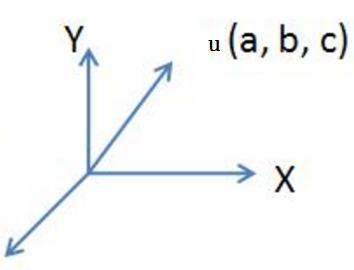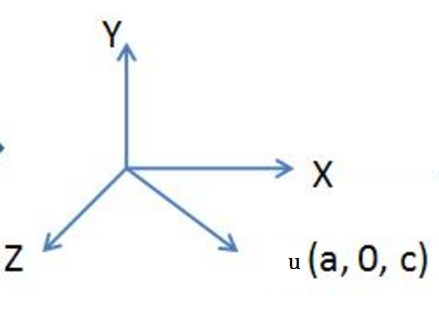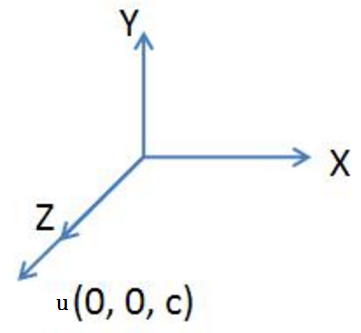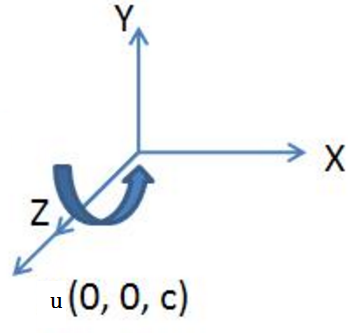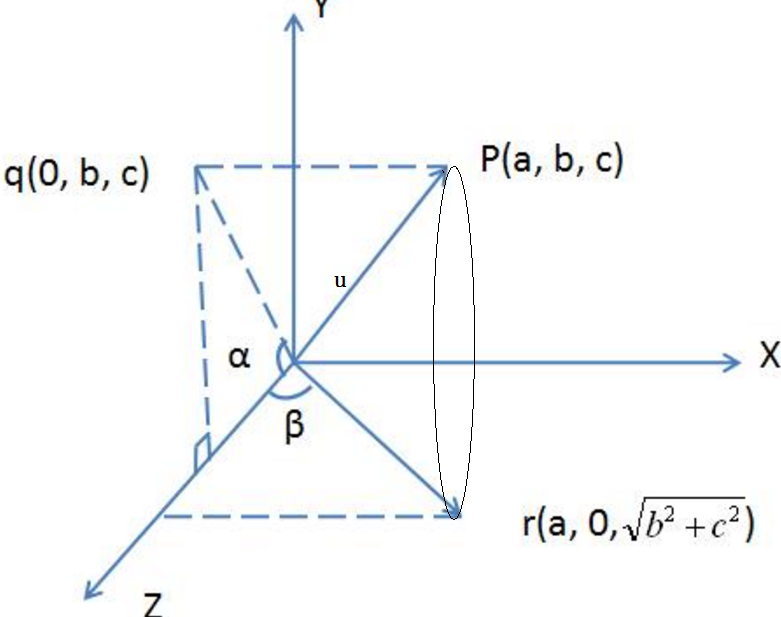10000cosθsinθ00sinθcosθ00001

cosα=c(b2+c2)

sinα=b(b2+c2)

10000c(b2+c2)b(b2+c2)00b(b2+c2)c(b2+c2)00001

cosθ0sinθ00100sinθ0cosθ00001

cosβ=(b2+c2)(a2+b2+c2)

sinβ=a(a2+b2+c2)

(b2+c2)(a2+b2+c2)0a(a2+b2+c2)00100a(a2+b2+c2)0(b2+c2)(a2+b2+c2)00001

cosθsinθ00sinθcosθ0000100001

Ry(β)=(b2+c2)(a2+b2+c2)0a(a2+b2+c2)00100a(a2+b2+c2)0(b2+c2)(a2+b2+c2)00001

Rx(α)=10000c(b2+c2)b(b2+c2)00b(b2+c2)c(b2+c2)00001

MR=Rx(α)Ry(β)Rz(θ)Ry(β)Rx(α)=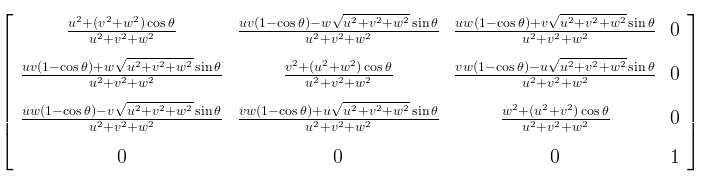(注：式中的(u,v,w)对应上文中向量(a,b,c)，公式我自己笔算过，为了减少编辑公式的时间（使用LaTex编辑太繁琐，因此找了一张公式的图片贴在此处)